Next: Gaussian approximation of the Up: Inferring numerical values of Previous: Hierarchical modelling and hyperparameters

## From Bayesian inference to maximum-likelihood and minimum chi-square model fitting

Let us continue with the case in which we know so little about appropriate values of the parameters that a uniform distribution is a practical choice for the prior. Equation (52) becomes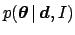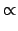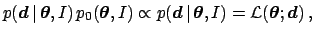(61)

where, we recall, the likelihood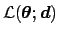is seen as a mathematical function of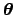, with parameters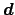.

The set ofthat is most likely is that which maximizes, a result known as the maximum likelihood principle. Here it has been obtained again as a special case of a more general framework, under clearly stated hypotheses, without need to introduce new ad hoc rules. Note also that the inference does not depend on multiplicative factors in the likelihood. This is one of the ways to state the likelihood principle, ideally desired by frequentists, but often violated. This principle' always and naturally holds in Bayesian statistics. It is important to remark that the use of unnecessary principles is dangerous, because there is a tendency to use them uncritically. For example, formulae resulting from maximum likelihood are often used also when non-uniform reasonable priors should be taken into account, or when the shape ofis far from being multi-variate Gaussian. (This is a kind of ancillary default hypothesis that comes together with this principle, and is the source of the often misused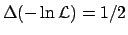' rule to determine probability intervals.)

The usual least squares formulae are easily derived if we take the well-known case of pairs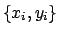(the genericstands for all data points) whose true values are related by a deterministic functionand with Gaussian errors only in the ordinates, i.e. we consider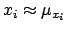. In the case of independence of the measurements, the likelihood-dominated result becomes,(62)

or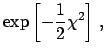(63)

where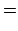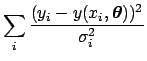(64)

is called chi-square,' well known among physicists. Maximizing the likelihood is equivalent to minimizing, and the most probable value ofis easily obtained (i.e. the mode indicated with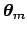), analytically in easy cases, or numerically for more complex ones.

As far as the uncertainty inis concerned, the widely-used evaluation of the covariance matrix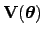(see Sect. 5.6) from the Hessian,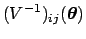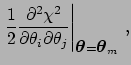(65)

is merely consequence of an assumed multi-variate Gaussian distribution of, that is a parabolic shape of(note that the' rule, and the from this rule resulting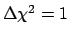rule,' has the same motivation). In fact, expanding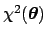in series around its minimum, we have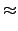(66)

where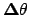stands for the the set of differences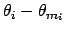and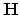is the Hessian matrix, whose elements are given by twice the r.s.h. of Eq. (65). Equation (63) becomes then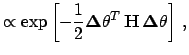(67)

which we recognize to be a multi-variate Gaussian distribution if we identify. After normalization, we get finally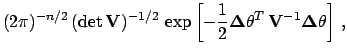(68)

with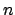equal to the dimension ofand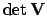indicating the determinant of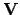. Holding this approximation,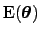is approximately equal to. Note that the result (68) is exact when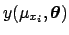depends linearly on the various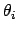.

In routine applications, the hypotheses that lead to the maximum likelihood and least squares formulae often hold. But when these hypotheses are not justified, we need to characterize the result by the multi-dimensional posterior distribution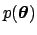, going back to the more general expression Eq. (52).

The important conclusion from this section, as was the case for the definitions of probability in Sect. 3, is that Bayesian methods often lead to well-known conventional results, but without introducing them as new ad hoc rules as the need arises. The analyst acquires then a heightened sense of awareness about the range of validity of the methods. One might as well use these recovered' methods within the Bayesian framework, with its more natural interpretation of the results. Then one can speak about the uncertainty in the model parameters and quantify it with probability values, which is the usual way in which physicists think.Next: Gaussian approximation of the Up: Inferring numerical values of Previous: Hierarchical modelling and hyperparameters
Giulio D'Agostini 2003-05-13